# Schwarzschild equation of motion: initial conditions

• I
• Yukterez
In summary,The Schwarzschild equation of motion, where coordinate length is differentiated by proper time is as far as I know.f

#### Yukterez

The Schwarzschild equation of motion, where coordinate length is differentiated by proper time is as far as I know

$$r''(t) = -\frac{G\cdot M}{r(t)^2} + r(t)\cdot{\theta}'(t)^2 - \frac{3\cdot G\cdot M\cdot{\theta}'(t)^2}{c^2}$$
$${\theta}''(t) = -2\cdot r'(t)/r(t)\cdot{\theta}'(t)$$

Now the question is, how do I choose my initial conditions for the angular and the radial initial velocities, for example, if I want to test the orbit of a test particle close to the speed of light $v_0=0.999c$ at the photon sphere $r_0=1.5r_s$?

I am not sure if my approach is correct, but when I try

$$\dot{\theta}_0\cdot r_0 = \frac{\xi_0}{ \color{red}{\sqrt{ 1-v_0^2/c^2}}\cdot \color{blue}{\sqrt{1-r_s/r_0}}}$$

with $\xi_0$ as the transversal component of $v_0$ and $\kappa_0$ as the radial component:

$$\dot{r}_0\ = \frac{\kappa_0\cdot \color{blue}{\sqrt{1-r_s/r_0}}}{ \color{red}{\sqrt{ 1-v_0^2/c^2}}}$$

so that $\xi = cos(\varphi_0), \kappa = sin(\varphi_0)$ with $\varphi$ as the launching angle from the shell, the orbits look like I would expect them, at least in the transversal direction: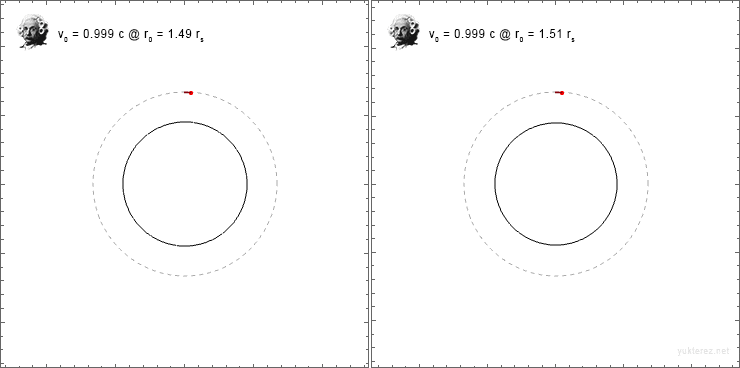But since radial and transverse directions have the factor $\color{blue}{\sqrt{1-r_s/r_0}}$ on different sides of the fraction, the inital angle is not conserved.

So are my initial conditions even correct, and if not, how would one transform the radial and the transversal components of the initial velocity $v_0$ to $\dot{r}(0)$ and $\dot{\varphi}(0)$?
_____________________________________________________

If it is of interest, the code for the plot is in Mathematica Syntax
Code:
G = 1; M = 1; c = 1; rs = 2 G M/c^2; T = tMax G M/c^3;

r0 = 151/100 rs; vo = 999/1000 c; φ = Pi/2; θ0 = 0; tMax = 2;
vr0 = vo Cos[φ]/j*k; vθ0 = vo/r0 Sin[φ]/j/k;
d1 = T/10; d2 = d1; wp = 30; step = T/200;

j = Sqrt[1 - vo^2/c^2];
k = Sqrt[1 - rs/r0];

sol = NDSolve[{
r''[t] == -((G M)/r[t]^2) + r[t] θ'[t]^2 - (3 G M)/c^2 θ'[t]^2,
r' == vr0,
r == r0,
θ''[t] == -((2 r'[t] θ'[t])/r[t]),
θ' == vθ0,
θ == θ0,
τ'[t] == Sqrt[c^2 r[t] + r[t] r'[t]^2 - c^2 rs + r[t]^3 θ'[t]^2 - r[t]^2 rs θ'[t]^2]/(c Sqrt[r[t] - rs] Sqrt[1 - rs/r[t]]),
τ == 0
}, {r, θ, τ}, {t, 0, T}, WorkingPrecision -> wp,
MaxSteps -> Infinity, Method -> Automatic,
InterpolationOrder -> All];

t[ξ_] :=
Quiet[χ /.
FindRoot[
Evaluate[τ[χ] /. sol][] - ξ, {χ, 0},
WorkingPrecision -> wp, Method -> Automatic]];
Τ := Quiet[t[ι]];

x[t_] := (Sin[Evaluate[θ[t] /. sol]] Evaluate[r[t] /. sol])[]
y[t_] := (Cos[Evaluate[θ[t] /. sol]] Evaluate[r[t] /. sol])[]

s[text_] := Style[text, FontSize -> font];  font = 11;

(* Eigenzeit, Celerity *) Do[Print[
Rasterize[Grid[{{Show[Graphics[{
{Black, Circle[{0, 0}, rs]},
{Lighter[Gray], Dashed, Circle[{0, 0}, r0]}},
Frame -> True, ImageSize -> 400, PlotRange -> 2 r0],
Graphics[{PointSize[0.01], Red, Point[{x[т], y[т]}]}],
ParametricPlot[{x[η], y[η]}, {η, 0, т},
ColorFunction -> Function[{x, y, η},
Hue[0.85, 1, 0.5, Max[Min[(-т + (η + d1))/d1, 1], 0]]],
ColorFunctionScaling -> False],
ParametricPlot[{x[η], y[η]}, {η, 0, т},
ColorFunction -> Function[{x, y, η},
Hue[0, 1, 0.5, Max[Min[(-т + (η + d2))/d2, 1], 0]]],
ColorFunctionScaling -> False]]},
{Grid[{
{"   ", s["proper time"], " = ", s[N[т, 8]], s["GM/c³"]},
{"   ", s["coordinate time"], " = ", s[N[Evaluate[τ[т] /. sol][], 8]], s["GM/c³"]},
{"   ", s["time dilation"], " = ", s[N[Evaluate[τ'[т] /. sol][], 8]], s["dτ/dt"]},
{"   ", s["angle"], " = ", s[N[Evaluate[(θ[т] /. sol) 180/Pi][], 8]], s["grad"]},
{"   ", s["radial distance"], " = ", s[N[Evaluate[r[т] /. sol][], 8]], s["GM/c²"]},
{"   ", s["x-Axis"], " = ", s[N[x[т], 8]], s["GM/c²"]},
{"   ", s["y-Axis"], " = ", s[N[y[т], 8]], s["GM/c²"]}
}, Alignment -> Left]}}, Alignment -> Left]]
], {т, step, T, step}]

Now the question is, how do I choose my initial conditions for the angular and the radial initial velocities, for example, if I want to test the orbit of a test particle close to the speed of light $v_0=0.999c$ at the photon sphere $r_0=1.5r_s$?
Finding initial conditions for these simulations is not trivial; I have devoted whole programs (some longer than the simulation itself) just to deriving useful sets. Coordinates and velocities are not particularly intuitive or useful for this purpose. For the Schwarzschild case (also Kerr, but that's for another day) you can use the constants of motion E and L (see equation 7.49 in Carroll for a circular orbit) and translate these into velocities when you initialize your solution.

I have provided links here many times to source code that does all this so I won't repeat it this time, but search for my posts regarding orbits if you are interested, it will save you a lot of time. I can probably help if you have a more specific question.

•vanhees71
Now the question is, how do I choose my initial conditions for the angular and the radial initial velocities, for example, if I want to test the orbit of a test particle close to the speed of light $v_0=0.999c$ at the photon sphere $r_0=1.5r_s$?

On further reflection it occurs that I might be able to help more with this, if you want to test out an orbit like the one you described. Let c = G = M = ##\mu## = 1. Then you should be able to get an orbit close to the photon sphere if you set:

a = 0.0
r = 3.001
E = 18.272630817366
L = 94.899952581663

You should get an unstable orbit where:

v = 0.999833 (##\gamma## = 54.781)

Hope that helps (I set this up on the JavaScript simulator, you can use it as an independent check for your stuff - or let me know if I've got something wrong!).

Last edited:
•edguy99
I came to the conclusion that the right way to transform the v₀ into the initial conditions for the equation of state, r'(0) and θ'(0), must be

$$\dot{\theta}(0)\cdot r(0) = \frac{{v\perp}_0}{ \color{red}{\sqrt{ 1-v_0^2/c^2}}}$$
$$\dot{r}(0) = \frac{{v\parallel}_0\cdot \color{blue}{\sqrt{1-r_s/r_0}}}{ \color{red}{\sqrt{ 1-v_0^2/c^2}}}$$

When I convert the initial velocity components by that factors I get the same time dilation with the differential

$${\tau}'(t) = \frac{\sqrt{c^2\cdot r(t)-c^2\cdot r_s +r(t)\cdot r'(t)^2- r_s\cdot r(t)^2\cdot \theta '(t)^2+r(t)^3\cdot \theta '(t)^2}}{c\cdot \sqrt{r(t)- r_s } \cdot\sqrt{1-\frac{ r_s }{r(t)}}}$$

as I get it with

$$\frac{1}{\sqrt{1-v_0^2/c^2}} \cdot \frac{1}{\sqrt{1-r_s/r_0}}$$

see the test calculation where Out matches Out: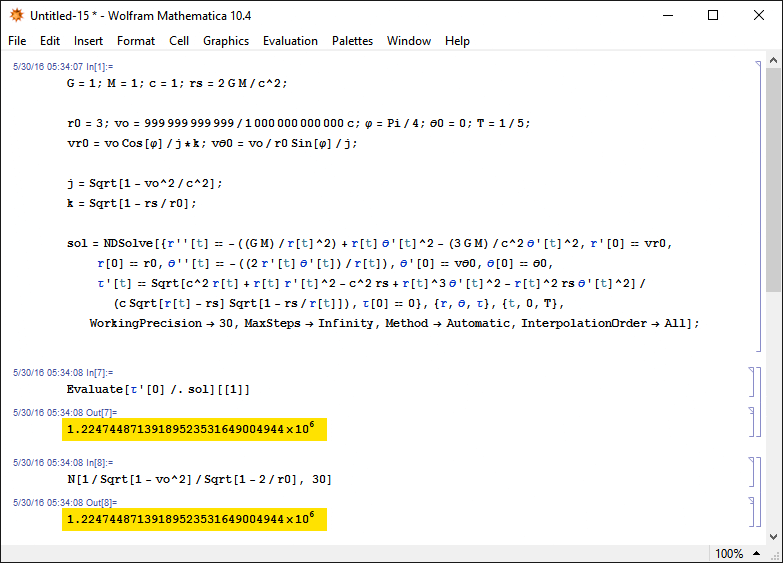m4r35n357 said:
r = 3.001
v = 0.999833 (γ = 54.781)
How did you obtain that value? When I use the formula √(GM/r0)/√(1-rs/r0) I get v0 = 0.9995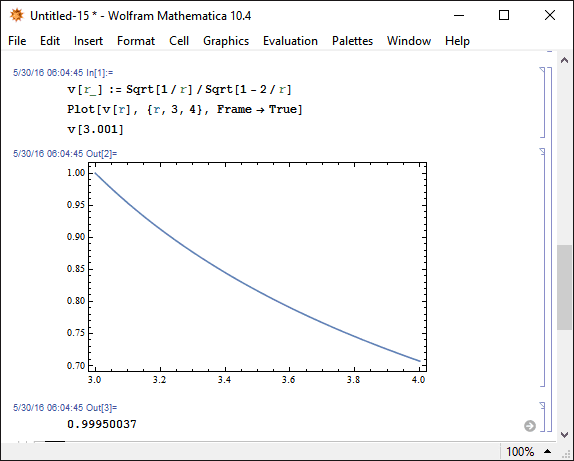I just logged on to post that I do not get

I am not sure if my approach is correct, but when I try

$$\dot{\theta}_0\cdot r_0 = \frac{\xi_0}{ \color{red}{\sqrt{ 1-v_0^2/c^2}}\cdot \color{blue}{\sqrt{1-r_s/r_0}}}$$

with $\xi_0$ as the transversal component of $v_0$ and $\kappa_0$ as the radial component:

$$\dot{r}_0\ = \frac{\kappa_0\cdot \color{blue}{\sqrt{1-r_s/r_0}}}{ \color{red}{\sqrt{ 1-v_0^2/c^2}}}$$

so that $\xi = cos(\varphi_0), \kappa = sin(\varphi_0)$ with $\varphi$ as the launching angle from the shell

When I logged on, I saw

I came to the conclusion that the right way to transform the v₀ into the initial conditions for the equation of state, r'(0) and θ'(0), must be

$$\dot{\theta}(0)\cdot r(0) = \frac{{v\perp}_0}{ \color{red}{\sqrt{ 1-v_0^2/c^2}}}$$
$$\dot{r}(0) = \frac{{v\parallel}_0\cdot \color{blue}{\sqrt{1-r_s/r_0}}}{ \color{red}{\sqrt{ 1-v_0^2/c^2}}}$$

This is what I get.

•Yukterez
This is what I get.
Very fine, then the first problem is solved, and only one question remains.

Because the blue gravitational factor is only in the radial direction it seems that angles are not preserved, for example, if someone throws a ball with 45° locally, the initial angle seems flatter for a coordinate observer at infinity (that's what I suspect)? Or do I have to split the components in a way that the angle is preserved (I don't think so)?

My guess is that if I want to switch from proper circumference to proper radial distance (since the factor is not 2π anymore in Schwarzschild coordinates) I'd have to do something like multiply the old r coordinate with rs+∫(1/√(1-rs/R), R=rs..r) on a Plot?

Last edited:
How did you obtain that value? When I use the formula √(GM/r0)/√(1-rs/r0) I get v0 = 0.9995

I don't see how the "potential" (blue) factor can produce a speed. I use the "kinetic" (red) factor:

##\gamma = \sqrt { \frac {1} {1 - v^2}} ##, so that

##v = \sqrt {1 - \frac {1 } { \gamma^2} }## (by ##v## I really mean ##v / c##!)

where ##\gamma = \frac {dt} {d\tau}## from the simulation to give me that value of ##v##.

Caveat:

We are using different methods to simulate and I must admit I don't fully understand your equations of motion. At first I thought they were the geodesic equations but I don't recognize the Christoffel symbols so now I'm not so sure.

Anyway, I think the ##t## in your equations is what I would refer to as ##\tau##, and ##\theta## is what I would refer to as ##\phi##. You would need to solve for ##t## (using another geodesic equation) and extract the intermediate ##\frac {dt} {d\tau}## in order to use my formula.

Are any of my assumptions mistaken?

Last edited:
Anyway, I think the ##t## in your equations is what I would refer to as ##\tau##, and ##\theta## is what I would refer to as ##\phi##. You would need to solve for ##t## (using another geodesic equation) and extract the intermediate ##\frac {dt} {d\tau}## in order to use my formula.

This is what I think, too. Maybe Yukterez has a math background, Mathematicians and physicists tend to interchange the roles of ##\phi## and ##\theta## in spherical coordinates.

I don't see how the "potential" (blue) factor can produce a speed. I use the "kinetic" (red) factor:

##\gamma = \sqrt { \frac {1} {1 - v^2}} ##, so that

##v = \sqrt {1 - \frac {1 } { \gamma^2} }## (by ##v## I really mean ##v / c##!)

where ##\gamma = \frac {dt} {d\tau}## from the simulation to give me that value of ##v##.

You're using ##\gamma## with respect to infinity, while Yukterez is using a ##\gamma## generated by a local physical velocity.

Suppose I stand on platform that uses a powerful rocket to keep it hovering constant ##r_0## and ##\theta## (i.e., ##\phi## for physics books). I then throw a ball that has initial speed ##v_0## (and that makes an initial angle ##\varphi## with respect to the platform). Then,

$$\gamma = \frac{1}{\sqrt{1 - v_0^2}} = \frac{dt}{d\tau} \sqrt{1 - \frac{2GM}{c^2 r_0}}.$$

When I get to work at about 9 Pacific time, I am going to have a look at Yukterez's equations of motion.

Last edited:
Here is the updated code (Mathematica Syntax), where θ is the longitude (the plane can always be rotated so that the lattitude is fixed), and φ the launching angle from the observers shell: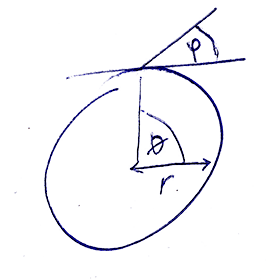Code:
G = 1; M = 1; c = 1; rs = 2 G M/c^2;

r0 = 151/100 rs; vo = 999/1000 c; φ = 0; θ0 = 0; tMax = 2; T = tMax G M/c^3;
vr0 = vo Sin[φ]/j*k; vθ0 = vo/r0 Cos[φ]/j;
d1 = T/10; d2 = d1; wp = 30; step = T/200;

j = Sqrt[1 - vo^2/c^2];
k = Sqrt[1 - rs/r0];

sol = NDSolve[{
r''[t] == -((G M)/r[t]^2) + r[t] θ'[t]^2 - (3 G M)/c^2 θ'[t]^2,
r' == vr0,
r == r0,
θ''[t] == -((2 r'[t] θ'[t])/r[t]),
θ' == vθ0,
θ == θ0,
τ'[t] == Sqrt[c^2 r[t] + r[t] r'[t]^2 - c^2 rs + r[t]^3 θ'[t]^2 - r[t]^2 rs θ'[t]^2]/(c Sqrt[r[t] - rs] Sqrt[1 - rs/r[t]]),
τ == 0
}, {r, θ, τ}, {t, 0, T}, WorkingPrecision -> wp,
MaxSteps -> Infinity, Method -> Automatic,
InterpolationOrder -> All];

t[ξ_] :=
Quiet[χ /.
FindRoot[
Evaluate[τ[χ] /. sol][] - ξ, {χ, 0},
WorkingPrecision -> wp, Method -> Automatic]];
Τ := Quiet[t[ι]];

x[t_] := (Sin[Evaluate[θ[t] /. sol]] Evaluate[r[t] /. sol])[]
y[t_] := (Cos[Evaluate[θ[t] /. sol]] Evaluate[r[t] /. sol])[]

s[text_] := Style[text, FontSize -> font];  font = 11;

(* proper time *) Do[Print[
Rasterize[Grid[{{Show[Graphics[{
{Black, Circle[{0, 0}, rs]},
{Lighter[Gray], Dashed, Circle[{0, 0}, r0]}},
Frame -> True, ImageSize -> 400, PlotRange -> 2 r0],
Graphics[{PointSize[0.01], Red, Point[{x[т], y[т]}]}],
ParametricPlot[{x[η], y[η]}, {η, 0, т},
PlotStyle->{LightGray}],
ParametricPlot[{x[η], y[η]}, {η, 0, т},
ColorFunction -> Function[{x, y, η},
Hue[0, 1, 0.5, Max[Min[(-т + (η + d2))/d2, 1], 0]]],
ColorFunctionScaling -> False]]},
{Grid[{
{" ", s["proper time"], " = ", s[N[т, 8]], s["GM/c³"]},
{" ", s["coordinate time"], " = ", s[N[Evaluate[τ[т] /. sol][], 8]], s["GM/c³"]},
{" ", s["time dilation"], " = ", s[N[Evaluate[τ'[т] /. sol][], 8]], s["dτ/dt"]},
{" ", s["angle"], " = ", s[N[Evaluate[(θ[т] /. sol) 180/Pi][], 8]], s["degree"]},
{" ", s["radial distance"], " = ", s[N[Evaluate[r[т] /. sol][], 8]], s["GM/c²"]},
{" ", s["x-Axis"], " = ", s[N[x[т], 8]], s["GM/c²"]},
{" ", s["y-Axis"], " = ", s[N[y[т], 8]], s["GM/c²"]}
}, Alignment -> Left]}}, Alignment -> Left]]
], {т, step, T, step}]

(* coordinate time *) Do[Print[
Rasterize[Grid[{{Show[Graphics[{
{Black, Circle[{0, 0}, rs]},
{Lighter[Gray], Dashed, Circle[{0, 0}, r0]}},
Frame -> True, ImageSize -> 400, PlotRange -> 2 r0],
Graphics[{PointSize[0.01], Red, Point[{x[Τ], y[Τ]}]}],
ParametricPlot[{x[η], y[η]}, {η, 0, Τ},
PlotStyle->{LightGray}],
ParametricPlot[{x[η], y[η]}, {η, 0, Τ},
ColorFunction ->
Function[{x, y, η},
Hue[0, 1, 0.5, Max[Min[(-Τ + (η + d2))/d2, 1], 0]]],
ColorFunctionScaling -> False]]},
{Grid[{
{" ", s["proper time"], " = ", s[N[Τ, 8]], s["GM/c³"]},
{" ", s["coordinate time"], " = ", s[N[ι, 8]], s["GM/c³"]},
{" ", s["time dilation"], " = ", s[N[Evaluate[τ'[Τ] /. sol][], 8]], s["dτ/dt"]},
{" ", s["angle"], " = ", s[N[Evaluate[(θ[Τ] /. sol) 180/Pi][], 8]], s["degree"]},
{" ", s["radial distance"], " = ", s[N[Evaluate[r[Τ] /. sol][], 8]], s["GM/c²"]},
{" ", s["x-Axis"], " = ", s[N[x[Τ], 8]], s["GM/c²"]},
{" ", s["y-Axis"], " = ", s[N[y[Τ], 8]], s["GM/c²"]}
}, Alignment -> Left]}}, Alignment -> Left]]
], {ι, step, T, step}]

Last edited:
You're using ##\gamma## with respect to infinity, while Yukterez is using a ##\gamma## generated by a local physical velocity.
I spent a good bit of time looking at this, and coming up with more complex approaches to calculating v (with and without being divided by the local "c") involving ##g_{tt}##, but none seemed right (particularly wrt to the value being bounded between 0 and 1).

My pragmatic and empirical approach has been to look at the division of the speed with c (a light pulse instantaneously coincident with the particle and moving in the same direction), and assume the potential (position) terms cancel (so I am not really wrt to infinity any more?).

For example, all the cases I looked at of "knife-edge" orbits just outside the photon sphere for ##a## values of 1.0 (##r = 1.001##), 0.0 (##r = 3.001##) and -1.0 (##r = 4.001##) had ##v/c## tending to 1.0 (which seemed right). So pragmatically, if my answer is wrong I would expect the (adjusted for position-dependent potential, non-infinity) values of ##v## to be potentially either over or under 1.0. Neither seems right, so I kept the simpler solution, but I would appreciate comments on this assumption (I don't have the formal maths chops to make my reasoning any more rigorous).

If I am wrong and anyone knows of any references for calculating local ##v / c## in GR I would appreciate pointers, I couldn't find any.

NB my value of ##v## is purely due to post-processing of results, I would not be so cavalier about the simulation itself!

I spent a good bit of time looking at this, and coming up with more complex approaches to calculating v (with and without being divided by the local "c") involving ##g_{tt}##, but none seemed right (particularly wrt to the value being bounded between 0 and 1).

My pragmatic and empirical approach has been to look at the division of the speed with c (a light pulse instantaneously coincident with the particle and moving in the same direction), and assume the potential (position) terms cancel (so I am not really wrt to infinity any more?).

For example, all the cases I looked at of "knife-edge" orbits just outside the photon sphere for ##a## values of 1.0 (##r = 1.001##), 0.0 (##r = 3.001##) and -1.0 (##r = 4.001##) had ##v/c## tending to 1.0 (which seemed right). So pragmatically, if my answer is wrong I would expect the (adjusted for position-dependent potential, non-infinity) values of ##v## to be potentially either over or under 1.0. Neither seems right, so I kept the simpler solution, but I would appreciate comments on this assumption (I don't have the formal maths chops to make my reasoning any more rigorous).

If I am wrong and anyone knows of any references for calculating local ##v / c## in GR I would appreciate pointers, I couldn't find any.

NB my value of ##v## is purely due to post-processing of results, I would not be so cavalier about the simulation itself!

I will try to expand on all this once I get to work. Trying to get my daughter and her visiting cousins out the door to school right now,

m4r35n357 said:
So pragmatically, if my answer is wrong I would expect the (adjusted for position-dependent potential, non-infinity) values of v to be potentially either over or under 1.0. Neither seems right
For example, for a transversal initial motion right above the photon sphere at r=3GM/c²:
If you take the local velocity (proper distance differentiated by proper time) the v0 should be around c.
If you take the coordinate velocity (coordinate distances differentiated by coordiante time) it should be below c.
If you take the rapidity (coordinate distances differentiated by proper time) it should be above c.
In my equation of motion the latter is the case. That makes it very similar to Newtons equation, except the additional term - 3GM/c² θ'² in
r'' = -((G M)/r'²) + r θ'² - (3 G M)/c² θ'², θ''= -((2 r' θ')/r)
so I transform the initial velocity into the rapidity.
Then I differentiate coordinate time by proper time with
τ' = √[c² r + r r'² - c² rs + r³ θ'² - r² rs θ'²]/(c √[r - rs] √[1 - rs/r])
and finally get the positions at any given proper and coordinate time. From there I can easily transform into the system of any stationary shell observer at height R just by multiplying the coordinate time at infinity with √(1-rs/R).

Test:

When I place an observer at a shell at r0=10rs and let him throw a ball with an initial velocity of √(GM/r0) in an angle of 45° (measured locally) this ball approaches the horizon of the central mass after a revolution of 312.1 degrees, 434.323143 GM/c³ proper time and in coordinate time and shell time it only converges to r=rs, but never actually crosses it:

On the left (Newtonian) and on the right (Einsteinian) the initial proper velocity and angle as measured by the observer at r0=10rs is the same, so the rapidity and energy is higher in the right animation. What results do you get for this configuration on your Java simulator?

Last edited by a moderator:
For example, all the cases I looked at of "knife-edge" orbits just outside the photon sphere for ##a## values of 1.0 (##r = 1.001##), 0.0 (##r = 3.001##) and -1.0 (##r = 4.001##) had ##v/c## tending to 1.0 (which seemed right). So pragmatically, if my answer is wrong I would expect the (adjusted for position-dependent potential, non-infinity) values of ##v## to be potentially either over or under 1.0. Neither seems right, so I kept the simpler solution, but I would appreciate comments on this assumption (I don't have the formal maths chops to make my reasoning any more rigorous).

I am having trouble unpacking this paragraph. For example. what is ##a##?

I am having trouble unpacking this paragraph. For example. what is ##a##?
##a## is the black hole spin parameter, normalized to the mass. The ##r## values I quoted are for particle orbits just outside the photon spheres at each of the ##a## values. Each example is supposed to be "close" to the actual photon sphere, where the value of ##v/c = c/c## has to be 1.0 by definition. My internal sense of physics justice says that there should not be a discontinuity in velocities at the photon sphere, but I can't express this in a more formal way. What I can do is try going closer, and verify that ##v/c## approaches 1.0 in practice. So far my prejudices have been confirmed. Does that make things clearer?

As I said, this is just post-processing and I will gladly chase up any analysis that I can find online. So far I have not found anything that calculates ##v/c## in curved spacetime.

What results do you get for this configuration on your Java simulator?
Hmm, difficult to say as we are using different methods of setting up the problems! While I look at comparisons like this all the time, it is not trivial to set this up with the precise parameters you have given. I would need to set my radius to ##10 r_s## and experiment with the L factor until I measure (on the screen, and I have no protractor!) 45 degrees, so there would be no accurate figures to compare.

Can you give at least a brief outline how you derive your equations of motion, as I don't recognize them from the materials that I have read? Without understanding your equations, how you set the initial conditions (and you seem to have solved that problem already), and without Mathematica, I can't really follow what you are doing very well.

BTW did you try my simulator? It is HTML/JavaScript not Java ;)

I would need to set my radius to 10 rs and experiment with the L factor until I measure (on the screen, and I have no protractor!) 45 degrees, so there would be no accurate figures to compare.
I don't think that the angle on the screen would be the same as the angle the observer on the shell would measure, since the Schwarzschild coordinates show the correct circumference, but the expandet length (the conversion factor between radius and circumference is not 2π, so the angle on the screen would look flatter than for the shell observer).

Can you give at least a brief outline how you derive your equations of motion, as I don't recognize them from the materials that I have read? Without understanding your equations, how you set the initial conditions
The equation uses the regular Schwarzschild coordinates as defined for an observer at infinity, but the proper time of the falling particle. If the velocity is c the rapidity is infinite. This allows an almost Newtonian form.

BTW did you try my simulator? It is HTML/JavaScript not Java ;)
I tried, but I couldn't find the input parameters for the initial velocity and angle, but if you tell me how your input parameters are defined so I can convert my angle and velocity into them I'd love to! Do you work in cartesian, polar or spherical coordinates?

Last edited:
The equation uses the regular Schwarzschild coordinates as defined for an observer at infinity, but the proper time of the falling particle. If the velocity is c the rapidity is infinite.

I tried, but I couldn't find the input parameters for the initial velocity and angle!
Touche ;) We do seem to have diverged almost beyond the limits of compatibility. You seem to be invoking the local frame of the particle, whereas I am not using one (I don't expect anyone to be alive on it to measure angles!) so I don't really have anything to measure angles against.

As to the other matter, I just don't recognize those equations, in five years of looking at this I've never seen the Schwarzschild metric or its Christoffel symbols with a 3 in there. They look a bit like geodesic equations because of the second derivatives and velocity terms, but that 3 is a real spanner in the works. I really would like to understand what you are solving, not being funny here.

I might be able to compare with your results by varying the plummet until I match up the azimuthal angle of impact, I'll give that a go later..

I played around with your simulator and at least I found out how to input a circular orbit at the photon sphere: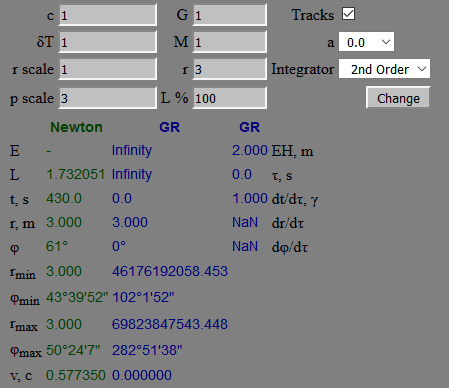This gives an orbital velocity in coordinate distances by coordinate time of v0=0.57735, so v0/√[1-rs/r0] with rs=2 and r0=3 is c locally. So far so good, this is not so hard to convert between our observers, just a factor of √[1-rs/r0] here and there. Now I just have to find out how to zoom in (if I set G=M=c=1 for easier numerics the plot gets really small) and give some initial radial velocity additional to the angular velocity. What parameter of yours is responsible for the radial velocity component, or alternatively the launching angle of the test particle?

What I also don't understand is why your E and L show infinity in this example when L<100%: Screenshot

As to the other matter, I just don't recognize those equations, in five years of looking at this I've never seen the Schwarzschild metric or its Christoffel symbols with a 3 in there.
The 3 is all over the place, see
https://www.astro.umd.edu/~miller/teaching/astr498/lecture10.pdf
http://christian.vonschultz.se/forelant/gravitation_and_cosmology/2008-11-20.pdf

Last edited:
##a## is the black hole spin parameter, normalized to the mass.

Okay, but this thread is about Shwarzschild, so let's keep ##a=0## everywhere.

For Yukterez's values of ##r_0##, ##v_0##, and ##\varphi_0##

$$E = \frac{\sqrt{1 - \frac{2}{r_0}}}{\sqrt{1 - v_0^2}}$$

$$L = \frac{v_0 r_0 \sin \varphi_0}{\sqrt{1 - v_0^2}},$$

How exactly is your L defined?
The program uses standard equations for circular orbits, and you tweak L% downwards to get interesting things to happen.
For Newton: ##L = \sqrt {r}##
For Kerr:
I would need to chase down the exact reference (there are standard formulae for circular orbits in Kerr spacetime), but here is the Js code:
Code:
    circular: function (r, a) {  // L and E for a circular orbit of r
var sqrtR = Math.sqrt(r);
var tmp = Math.sqrt(r * r - 3.0 * r + 2.0 * a * sqrtR);
this.L = (r * r - 2.0 * a * sqrtR + a * a) / (sqrtR * tmp);
this.E = (r * r - 2.0 * r + a * sqrtR) / (r * tmp);
},

As for the particle simulator, you can't go to 3.0 as it is a photon orbit. Try these settings to start with . . .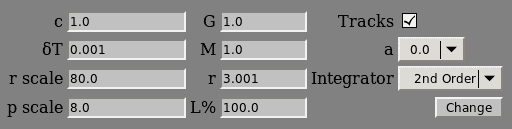On another note, have you tried GRorbits (Java)? I think it is more compatible with your initial conditions setting approach, and is good for checking basic operation. I used it while I only had one implementation.

Okay, but this thread is about Shwarzschild, so let's keep ##a=0## everywhere.
Point taken, but the program does really simulate Schwarzschild using Kerr with ##a = 0##.

BTW do you have any pointers for my velocity equation from post #8?

What I also don't understand is why your E and L show infinity in this example when L<100%: Screenshot
You can't do 3.0 because it's not a valid particle orbit, so the program is not working. You should be able to get quite close to 3.0 though.
The first is quoted without proof, and the second is . . . a bit complicated to take in (I'm not really a maths head, I do the minimum possible). I'll assume these are equivalent to the geodesic equations, but not using Christoffel symbols. As I mentioned before, I prefer first-order solutions, much simpler mathematics, and to be honest I spent most of my time working on all the integrators!

Point taken, but the program does really simulate Schwarzschild using Kerr with ##a = 0##.

BTW do you have any pointers for my velocity equation from post #8?

Call the "speed" that you use ##V##, and the speed that Yukterez and I use ##v##. Then,

$$E = \gamma_V \left( 1 - \frac{2}{r} \right) = \gamma_v \sqrt{1 - \frac{2}{r}},$$

which can be solved to give

$$v = \sqrt{1 -\left( 1 - V^2 \right) \left(1 - \frac{2}{r} \right)}$$

I don't know of a physical interpretation for ##V##, but ##v## has a simple interpretation in terms of hovering observers (also called shell observers). Suppose there is a hovering observer at each place in space, i.e., a different hovering observer for each ##r## and ##\phi## (##\theta## for Yukterez). Then the particle passes a series of hovering observers as it moves along its trajectory, and ##v## is the relative physical speed between the particle and each hovering observer that it visits.

Okay, but this thread is about Shwarzschild, so let's keep ##a=0## everywhere.

For Yukterez's values of ##r_0##, ##v_0##, and ##\varphi_0##

$$E = \frac{\sqrt{1 - \frac{2}{r_0}}}{\sqrt{1 - v_0^2}}$$

$$L = \frac{v_0 r_0 \sin \varphi_0}{\sqrt{1 - v_0^2}},$$

The expression for ##E## can be inverted to give

$$v = \sqrt{1 - \frac{1 - \frac{2}{r}}{E^2}}.$$

Thanks George.

Hmm, sorry but I'm not sure I understand what you are telling me. Is one of the equations above the one I should be using instead of what I have in #8?

You can't do 3.0 because it's not a valid particle orbit
But one could still let the particle fall into the black hole or escape to infinity, just because an orbit is not stable it doesn't mean it can't be plotted!

On another note, have you tried GRorbits (Java)?
Unfortunately the new Java version, at least on Windows, won't open that program anymore, it closes as soon as I open it and Java doesn't even offer me to try it anyway.

But one could still let the particle fall into the black hole or escape to infinity, just because an orbit is not stable it doesn't mean it can't be plotted!
It's not a question of stability; all circular particle orbits between (just over) 3M and 6M are unstable. 3M and less is simply not allowed mathematically for particles.

Unfortunately the new Java version, at least on Windows, won't open that program anymore, it closes as soon as I open it and Java doesn't even offer me to try it anyway.
Did you try downloading and running locally? Mine still works on Ubuntu, do you have Linux VM anywhere? It really is quite a good program.

3M and less is simply not allowed mathematically for particles.
Why not? For example, imagine a shell observer who is hovering at r0=2.5M=1.25rs. Now let him throw a particle at a launching angle of 30° with an initial velocity of v=0.9c (relative to himself in his own reference frame). This particle crashes into the black hole after approximately 5GM/c³ of its own proper time. Here the Plots by coordinate time of an observer at infinity where you can see the particle slow down when it approaches the horizon: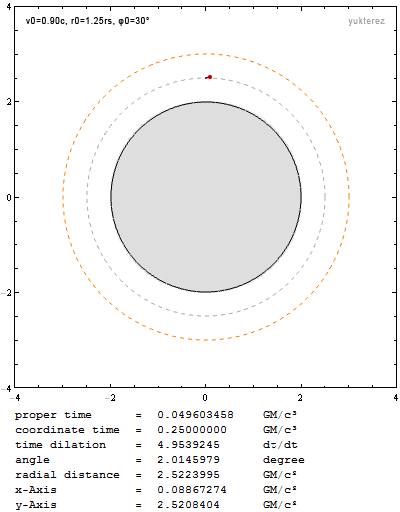When I tell the observer to increase the initial velocity to v0=0.95c, the particle escapes to infinity: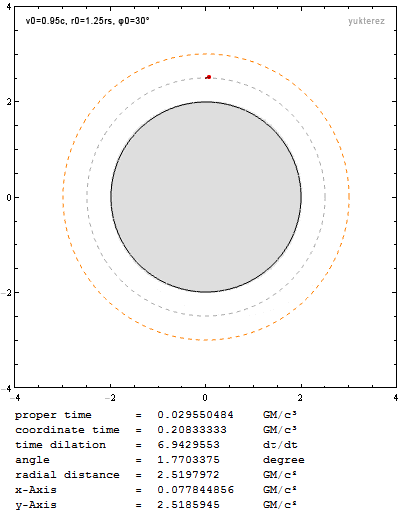So why shouldn't this be mathematically allowed? As long it is not at r0=rs or below, an observer can surely be a stationary shell observer, and he also can shine light and throw particles in any direction. What do you mean with "not allowed"?

Last edited:
So why shouldn't this be mathematically allowed? As long it is not at r0=rs or below, an observer can surely be a stationary shell observer, and he also can shine light and throw particles in any direction. What do you mean with "not allowed"?
Ahem, we are at cross purposes here, so let's rewind a bit. This started when you tried to set an initial circular orbit of 3.0, because my program sets its initial conditions via circular orbits. Circular orbits do not exist for particles at 3.0M or less. Yes you can hover (as long as you are "above" 2.0M), yes you can pass through the region inwards or outwards, yes you can plummet. But you cannot orbit. That is all I meant.

my program sets its initial conditions via circular orbits.
Now I get it, in this case it is of course mathematically not allowed to find a circular orbit at 3GM/c² and below.

Call the "speed" that you use ##V##, and the speed that Yukterez and I use ##v##.
Sorry George, I've left this stewing for a day in my mind, and I'm still not sure what you are telling me here. Am I supposed to be using the last equation (in your notation I was looking for ##V = something##)? BTW I don't have access to the ##v## that Yukterez uses so any expression containing that would involve rewriting my program. I reiterate that my ##V## is strictly an output of the program (I have access to ##\frac {d t} {d \tau}= \gamma##, ##\frac {d r} {d \tau}##, and ##\frac {d \phi} {d \tau}##, these and the metric components are my only ingredients), and not part of the simulation.

And I still don't understand what is wrong with ##V = \sqrt {1 - \frac {1 } { \gamma^2} }##. It seems to produce reasonable results under every situation that I can throw at it.

Just to be clear, I am looking for an expression which calculates ##v/c## (where c is the speed of a light pulse instantaneously in the same place and moving in the same direction) for a particle in curved spacetime. I think I already have it, so can you tell me what is wrong with it?

And I still don't understand what is wrong with ##V = \sqrt {1 - \frac {1 } { \gamma^2} }##.
What exactly are the equations of motion that you are using?

What exactly are the equations of motion that you are using?
I'm using equations 2 and 3 from this paper, simplified for equatorial motion. But as I've said a few times, I'm not using ##V## in the simulator, which I have verified to be correct.

My only question is about particle speed in GR, not about simulation.

My only question is about particle speed in GR, not about simulation.
Then the answer is easy. It is both true, either to say
"The light ray has a local velocity of v=c when it is entering the event horizon"
or
"The light ray has a coordinate velocity of V=0 when it freezes at the event horizon".
As long as one knows if you are talking about the motion with respect to local or coordinate time you can use both.

If you had to pick one and drop the other I would recommend the local velocity, because you can always transform from local to coordinate, but not always the other way around (in the limit of r=rs for example it is better to have a number that you can multiply with zero to get from the local system to the outer one, than to have a zero and divide it by zero to get from the outer system to the local one).

Off topic, but maybe also of interest:
In my simulation the input is local velocity, which is then converted to rapidity for the initial conditions in r and θ (which is the most effective way to process the actual equation of motion in an almost Newtonian formalism). Ιn the output the velocity is plotted either in proper or coordinate time invervals (v or V).

Last edited:
If you had to pick one and drop the other I would recommend the local velocity, because you can always transform from local to coordinate, but not always the other way around (in the limit of r=rs for example it is better to have a number that you can multiply with zero to get from the local system to the outer one, than to have a zero and divide it by zero to get from the outer system to the local one).

I don't have to pick one, I only have one, and that is the one I need.

That's the thing, I'm just not using a local frame at all, so I don't have, want or need a local velocity. It is frustrating that I am struggling so hard to make myself understood.

A particle has a coordinate velocity in the global coordinates. A pulse of light at the same point moving in the same direction has a velocity in the same global coordinates. I just want to divide the former by the latter, no more than that. I would like to know what proportion of light speed my particle is moving at. I have provided what I think is the answer, and have been trying in vain to solicit opinions on whether it is right and what I should be using if it is wrong.

Thanks for having a go, honestly, but I need an answer that does not mention a local frame or velocity, otherwise we are just going round in circles ;)